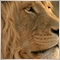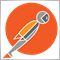# Number of Consecutive Sell Losses and Buy Losses163

Hi All

I am trying to get the consecutive number of losses from within my EA. The number does count up but when i get a win ,either on buy or sell the counter does not reset. Is there something wrong with my code?

```void BuyCountLosses()
{

for( cnt = OrdersHistoryTotal() - 1; cnt >= 0; cnt--)
{
sel =OrderSelect(cnt, SELECT_BY_POS, MODE_HISTORY);
if(OrderSymbol() == Symbol())
{
if (OrderProfit() < 0)
{
}
};

if (OrderProfit() > 0)
{
}
};

}
}

}

//++++++++++++++++++++++++++++++++++++++++++++++++++++++++++++++++++++++++++++++++++++++++++++++++++++++++++++++++
//++++++++++++++++++++++++++++++++++++++++++++++++++++++++++++++++++++++++++++++++++++++++++++++++++++++++++++++++
void SellCountLosses()
{
int  LossSell = 0;

for( cnt = OrdersHistoryTotal() - 1; cnt >= 0; cnt--)
{
sel =OrderSelect(cnt, SELECT_BY_POS, MODE_HISTORY);
if(OrderSymbol() == Symbol())
{
if ( OrderType() == OP_SELL){
if  (OrderProfit() < 0)
{
LossSell++;
}
}

if ( OrderType() == OP_SELL){
if  (OrderProfit() > 0)
{
LossSell=0;
}
}

}
}
TotalLossSell = LossSell ;

}```20207

1. Set the count to zero and start looping. If it's a loss, increment the count. If it's a win, exit the loop, you have your count already set.
2. Do not assume history is ordered by date, it's not.
Could EA Really Live By Order_History Alone? (ubzen) - MQL4 and MetaTrader 4 - MQL4 programming forum
Count how many lost orders from the last profit order - MQL4 and MetaTrader 4 - MQL4 programming forum163

whroeder1:
1. Set the count to zero and start looping. If it's a loss, increment the count. If it's a win, exit the loop, you have your count already set.
2. Do not assume history is ordered by date, it's not.
Could EA Really Live By Order_History Alone? (ubzen) - MQL4 and MetaTrader 4 - MQL4 programming forum
Count how many lost orders from the last profit order - MQL4 and MetaTrader 4 - MQL4 programming forum

Works perfectly now. Thanks

```void BuyCountLosses()
{

for( cnt = OrdersHistoryTotal() - 1; cnt >= 0; cnt--)
{
sel =OrderSelect(cnt, SELECT_BY_POS, MODE_HISTORY);
if(OrderSymbol() == Symbol())
{
if (OrderProfit() < 0)
{
}
};

if (OrderProfit() > 0)
{
break;
}
};

}
}

}

//++++++++++++++++++++++++++++++++++++++++++++++++++++++++++++++++++++++++++++++++++++++++++++++++++++++++++++++++
//++++++++++++++++++++++++++++++++++++++++++++++++++++++++++++++++++++++++++++++++++++++++++++++++++++++++++++++++
void SellCountLosses()
{
int  LossSell = 0;

for( cnt = OrdersHistoryTotal() - 1; cnt >= 0; cnt--)
{
sel =OrderSelect(cnt, SELECT_BY_POS, MODE_HISTORY);
if(OrderSymbol() == Symbol())
{
if ( OrderType() == OP_SELL){
if  (OrderProfit() < 0)
{
LossSell++;
}
}

if ( OrderType() == OP_SELL){
if  (OrderProfit() > 0)
{
break;
}
}

}
}
TotalLossSell = LossSell ;

}```2392

Simon Mungwira:

Works perfectly now. Thanks

You missed a step... you need to first sort the order history pool by close-time (see whroeder's #2), and then loop over it to count losses. That part isn't so easily done, but here is an example of how to do it.

```#include <Arrays\List.mqh>
class Deal : public CObject
{
public:
int      ticket;
datetime close_time;
double   profit;
Deal(int t,datetime ct,double p):ticket(t),close_time(ct),profit(p){}
virtual int Compare(const CObject *node,const int mode=0)const override
{
const Deal *other=node;
if(this.close_time > other.close_time) return -1; //sort descending
if(this.close_time < other.close_time) return 1;
return 0;
}
};

int consecutive_losses(int order_type)
{
CList deal_list;
for(int i=OrdersHistoryTotal()-1;i>=0;i--)
if(OrderSelect(i,SELECT_BY_POS,MODE_HISTORY) && OrderSymbol()==_Symbol && OrderType()==order_type)

deal_list.Sort(0);
int count = 0;
for(Deal *deal=deal_list.GetFirstNode(); deal!=NULL; deal=deal.Next())
if(deal.profit < 0.0)
count++;
else
break;
return count;
}```

...and then you would call it like...

```buy_losses = consecutive_losses(OP_BUY);
sell_losses = consecutive_losses(OP_SELL);```